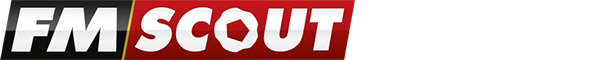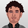On FM Scout you can chat about Football Manager in real time since 2011. Here are 10 reasons to join!

# 5 stars training in Football Manager 2011

Analysis of the latest coach formula in FM2011 to help you find the best coaches

By Updated on Jan 21, 2011   183843 views   2 commentsThis is the newest coach formula used in Football Manager 2011 to determine the stars coaches have in various training areas based on their attributes.

Strength:
Fitness * 9 + ddm * 2
>= 270 = 5 stars
>= 240 = 4.5 stars
>= 210 = 4 stars
>= 180 = 3.5 stars
>= 150 = 3 stars
>= 120 = 2.5 stars
>= 90 = 2 stars
>= 60 = 1.5 stars
>= 30 = 1 star
>= 0 = 0.5 stars

Aerobic:
Fitness * 9 + ddm * 2
>= 270 = 5 stars
>= 240 = 4.5 stars
>= 210 = 4 stars
>= 180 = 3.5 stars
>= 150 = 3 stars
>= 120 = 2.5 stars
>= 90 = 2 stars
>= 60 = 1.5 stars
>= 30 = 1 star
>= 0 = 0.5 stars

Goalkeeping - Shot Stopping:
Goalkeepers * 2 + ddm
>=90 = 5 stars
>=80 = 4.5 stars
>=70 = 4 stars
>=60 = 3.5 stars
>=50 = 3 stars
>=40 = 2.5 stars
>=30 = 2 stars
>=20 = 1.5 stars
>=10 = 1 star
>=0 = 0.5 stars

Goalkeeping - Handling:
Goalkeepers * 2 + ddm
>=90 = 5 stars
>=80 = 4.5 stars
>=70 = 4 stars
>=60 = 3.5 stars
>=50 = 3 stars
>=40 = 2.5 stars
>=30 = 2 stars
>=20 = 1.5 stars
>=10 = 1 star
>=0 = 0.5 stars

Tactics:
Tactical * 2 + ddm
>=90 = 5 stars
>=80 = 4.5 stars
>=70 = 4 stars
>=60 = 3.5 stars
>=50 = 3 stars
>=40 = 2.5 stars
>=30 = 2 stars
>=20 = 1.5 stars
>=10 = 1 star
>=0 = 0.5 stars

Ball Control:
Technical * 6 + Mental * 3 + ddm * 2
>= 270 = 5 stars
>= 240 = 4.5 stars
>= 210 = 4 stars
>= 180 = 3.5 stars
>= 150 = 3 stars
>= 120 = 2.5 stars
>= 90 = 2 stars
>= 60 = 1.5 stars
>= 30 = 1 star
>= 0 = 0.5 stars

Defending:
Defending * 8 + (Tactical + ddm) * 3
>=360 = 5 stars
>=320 = 4.5 stars
>=280 = 4 stars
>=240 = 3.5 stars
>=200 = 3 stars
>=160 = 2.5 stars
>=120 = 2 stars
>=80 = 1.5 stars
>=40 = 1 star
>=0 = 0.5 stars

Attacking:
Attacking * 6 + Tactical * 3 + ddm * 2
>= 270 = 5 stars
>= 240 = 4.5 stars
>= 210 = 4 stars
>= 180 = 3.5 stars
>= 150 = 3 stars
>= 120 = 2.5 stars
>= 90 = 2 stars
>= 60 = 1.5 stars
>= 30 = 1 star
>= 0 = 0.5 stars

Shooting:
Technical * 6 + Attacking * 3 + ddm * 2
>= 270 = 5 stars
>= 240 = 4.5 stars
>= 210 = 4 stars
>= 180 = 3.5 stars
>= 150 = 3 stars
>= 120 = 2.5 stars
>= 90 = 2 stars
>= 60 = 1.5 stars
>= 30 = 1 star
>= 0 = 0.5 stars

Key:
ddm: Determination + Discipline + Motivating
>= : equal to or greater than

Credits:
Thanks to BonzoLLM at The Dugout for this!

##### Your content on FM Scout

We are always looking for quality content creators, capable of producing insightful articles. Being published here means more exposure and recognition for you.

Do YOU have what it takes?

## Discussion: 5 stars training in Football Manager 2011

2 comments have been posted so far.

•Unfortunately this no longer works 100% for football manager 2014. They seem to have added another attribute to some of them. My assumption would be adaptability but I haven't tested it
•YES STAM!
###### FMS Chat–Stam
hey, just wanted to let you know that we have a fb style chat for our members. login or sign up to start chatting.